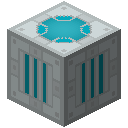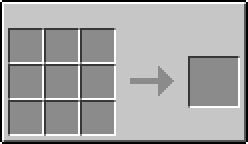TeleporterModIndustrialCraft 2
TypeMachine

The Teleporter is a machine in IndustrialCraft 2 that is used to instantly transport players, animals, and mobs over a distance to another Teleporter. The EU cost of the teleportation depends on the distance covered, and the weight of what is being teleported.

## Recipe## Usage

### Powering the Teleporter

Due to the large amount of EU used to teleport, it is not sufficient to connect a Teleporter to the EU network with Cables; not enough EU could be provided quickly enough. Instead, the Teleporter must be placed directly touching an EU storage unit: BatBoxes, MFE's, MFSU's, LESU's, IESU's, and AESU's all work. The EU cost of teleporting will be shared equally between any EU storage units that are adjacent to the Teleporter.

#### Calculating the EU Cost

The Teleporter uses a somewhat complicated formula for determining how many EU's are needed to perform one teleportation:This formula has two key variables- weight, and distance.

##### Weight Formula

The weight of an animal is always 500, and the weight of a mob is always 100. The weight of a player can be determined with the following formula:The pieces variable refers to the number of armor pieces that the player is wearing. The inv variable is determined by the formula,, for each stack of items in the player's inventory.

##### Example Calculation for Weight

Given a player that is wearing NanoSuit Boots for armor, and has in his inventory 64 Cobblestone, 3 Diamonds, and 6 Snowballs:##### Distance Formula

The calculation for distance is as follows:where x, y, and z are the respective end coordinate subtracted by the starting coordinate.

##### Example Calculation for Distance

If the first Teleporter is placed at (25, 60, -40), and the second Teleporter is placed at (40, 35, -30), the total distance is:##### Example Finished Calculation

Assuming the figures in the examples above, the total EU cost for one teleportation is: